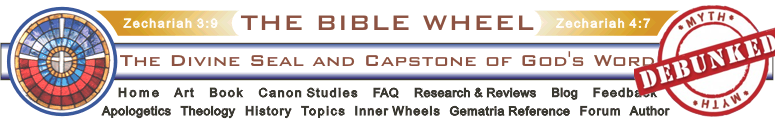Historical Archive of the Bible Wheel Site

The Bible Wheel had been debunked by its author.
Read all about it: Debunking Myself: What A Long Strange Trip It's Been

Recent Blog ArticlesNevertheless the foundation of God standeth sure, having this seal, The Lord knoweth them that are his. And, Let every one that nameth the name of Christ depart from iniquity.

II Timothy 2.19

The Holographic Generating set consists of three geometrically integrated Numbers: the Third Cube, 27, and the Fourth Hexagon/Star pair, 37/73.

A = 27    B = 37    C = 73

Many of the results in this table are derived in the introductory article. It is extremely important to note the simplicity of the GenSet expressions. This table shows that a single geometric meta-pattern runs through the entire holographic structure of the Holy Scripture, and that integrated with the Divine Names Jesus, YHVH, Elohim, and Alpha Omega, to name but a few. Glory!

 Fundamental Generating Set Identities A + B = 64 = Truth (, Aletheia) B - A = 10 [Base Ten] A + C = 100 = Ten Squared B + C = 110 = Foundation A + B + C = 137 = The God of Truth AB = 999 = Ten Cubed - 1 = In the beginning God (913 + 86) B2 = 1369 = Image of God (Ikon Theou) = And the Spirit of God moved upon the face of the waters Genesis 1.2 AB + 1 = 1000 = He Who Overcometh [1 John 5.5] B2 + AB = 2368 = JESUS CHRIST BC = Genesis 1.1 From Prime Composition = Sum(C) From Triangular Structure = Sum(B)+ 2AB From Triangular Substructure = A2 + AC + 1 From Self-reflection of GenSet BC + AB = Gen 1.1 + AB Gen 1.1 and John 1.1 connected to 3700 through distribution of GenSet. = John 1.1 + C = 100B = 3700 C2 + AB = Gen 1.1 + John 1.1 Sum of Gen 1.1 and John 1.1 form 112th Triangle that is also a very simple combination of all the elements of the GenSet = Sum(112)= Sum(C + 39) 100B -  C = John 1.1 John 1.1 and the Word result from very similar combinations of GenSet elements. 10B + C = THE WORD (443) 110B = B2 + BC This links to Foundation = 110 = B + C = Gen 1.1 + Image of God = John 1.1 + THE WORD A2 + AB = The Altar = 1728 = 123. Cf. Ezekiel 43.16: The Altar = 12 x 12 cubits! A2 + BC = 3430 This integrates with the HoloDec 48455 = 13 x 3430 + 1370          = 13(A2 + BC) + 10D = The light shineth in thedarkness John 1.5 15D = All things weremade by him Entire Prologue reflects value of the central claim. 195D = 13 x 15D 195D = John 1.1-5 624B = John 1.2-5 Sum is integrated with fundamental Divine Names and Attributes.  = 39 (YHVH EChD) x 592 (GODHEAD) = 26 (YHVH) x 888 (JESUS) = 13 x 48B (see next) 48B = And God said, Let there be light: and there was light. And God saw the light, that it was good: Gen 1.3f from the First Day Holograph = And God saw the light, that it was good: and God divided the light from the darkness. Gen 1.4 10C = 730 = A Discerner = Sharp, Eyes, etc. This, and the following 5 identites are from the Logos Holograph [Heb. 4.12] 60B + 6C = For the Word of God is Living Heb. 4.12Cf. 443 = 10B + C, similar form of John 1.1 60C = and sharper than any twoedged sword Heb. 4.12 77C = piercing even to the dividing asunder of soul and spirit Heb 4.12 CD = and sharper than any twoedged sword, piercing even to the dividing asunder of soul and spirit Heb 4.12 = 10001 = 104 + 1 = 73 x 137 = Genesis 1.1 + 7300 AD = a discerner of the thoughts and intents of the heart Heb 4.12 = Genesis 1.1 + AB - 1 = John 1.1 + C -1 38C = the Word was made flesh and dwelt amongst us. [John 1.14 - the Grace Manifest Holograph] 36B = 1332 = ALPHA OMEGA = Exodus 20.1: "And God spake all these words, saying," This is the introduction to the HoloDec. = 2Sum(B) 12D = And thou shalt love the LORD thy God with all thine heart, and with all thy soul, and with all thy might. Deut. 6.5, from the extended Unity Holograph.

Copyright © 2021 Richard Amiel McGough All Rights Reserved
Privacy Policy   |   Site Map   |   Contact: richard@biblewheel.com